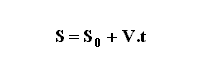Science

Kinematics

 Show figure Uniform Motion   Uniformly Accelerated Motion Calculate:   Final Position (S) Elapsed Time (t) Velocity (V) Initial Position (S0) =    m S = mt  = s V = m/sVo = m/s a  = m/s2To Memory 01 Memory 02 Memory 03 Memory 04 Memory 05 Memory 06 Replacing Adding Subtracting Multiplying Dividing
 Configurations Maximum of decimal places Units:     International System (SI)       English Units Impression Page Title (Optional) Comments to be printed (Optional)